# Sv-Plots and Testing Hypotheses

## Introduction

Sample variance is one of the measures of dispersion of the data. Sample variance plots (Sv-plots) introduced by , provide an appealing graphical tools which illustrate the contribution of squared deviation from each observation in the sample to make the sample variance. Further, these plots found to be revealing important characteristics of the population such as symmetry, skewness and outliers. Further, one version of Sv-plots innovates a graphical method for making decision on hypothesis testing over Population mean.

## Sv-plots

Two versions of Sv-plots, Sv-plot1 and Sv-plot2, provide two graphical illustrations of squared deviations in sample variance. To create these versions, let svplots package be installed first along with ggplot2 and stats in R.

library(svplots)
library(ggplot2)
library(stats)

In svplots package, functions svplot1 and svplot2 provide Sv-plot1 and Sv-plot2 while test1mu, test1musm, test2mu and test2musm lead to make the decision on the hypothesis testing over single and two population means with the data and summary statistics respectively.

### Sv-plot1

The first version of sample variance plots, Sv-plot1, illustrates each squared deviation by the area of a regular rectangle, equivalently by a square with the side equal to the deviation. Besides, for the purpose of detecting outliers in the data, Sv-plot1 includes two bounds (dash squares) generated at $$Q_1-1.5IQR$$ and $$Q_3+1.5IQR$$, where $$Q_1$$, $$Q_3$$ and $$IQR$$ are 1st quartile, 3rd quartile and interquartile range ($$IQR=Q_3-Q_1$$) respectively. Examples 1 and 2 display Sv-plot1 for two simulated datasets provided by svplot1.

#### Example 1

set.seed(2021)
X<-matrix(rnorm(50,mean=2,sd=5))
svplot1(X,title="Sv-plot1",xlab="x",lbcol="grey5",lscol="grey60",rbcol="grey45",rscol="grey75")
#> $Summary #> Sample_Size Average Left_SS Right_SS Sample_Variance #> 1 50 2.0794 800.0535 771.9212 32.0811 #> #>$Svplot1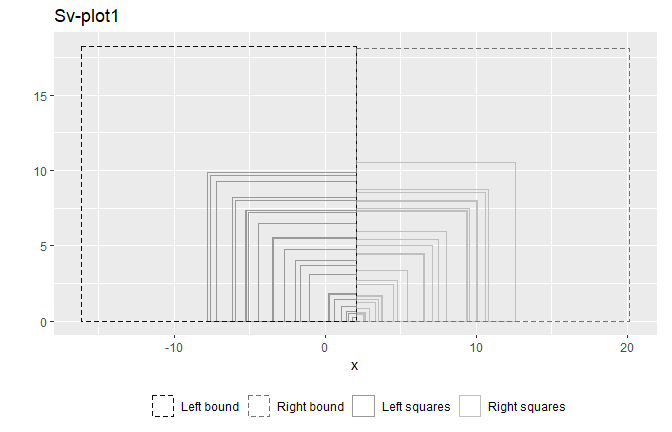Figure 1. Sv-plot1 illustrates that both left and right squares are evenly distributed about the mean indicating a symmetry of the data distribution.

#### Example 2

set.seed(5)
X <- matrix(rbeta(50,shape1=10,shape2=2))
g<-svplot1(X,title="Sv-plot1",lbcol="grey5",lscol="grey60",rbcol="grey45",rscol="grey75")
g1=g$Svplot1+theme(panel.grid.minor= element_blank(), panel.grid.major= element_blank(), legend.position = 'none', panel.background = element_rect(fill = "transparent",color = "black")) g$Summary
#>   Sample_Size Average Left_SS Right_SS Sample_Variance
#> 1          50  0.8316  0.4502   0.1854           0.013
g1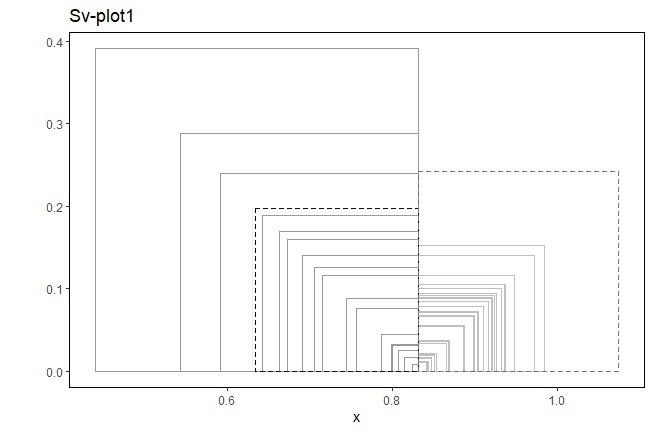Figure 2. Existence of many left large squares in Sv-plot1 indicates a skewed left distribution. Three squares outside the left dashed square correspond to outliers.

## Sv-plot2

The second version of sample variance plots, Sv-plot2, illustrates value of the squared deviation against each data value. The two bounds, horizontal dash-half-lines placed at the squared deviations evaluated at $$Q_1-1.5IQR$$ and $$Q_3+1.5IQR$$, identify the outliers in the dataset. Example 3 and 4 display Sv-plot2 for two simulated datasets provided by svplot2.

### Example 3

set.seed(10)
X<-matrix(rf(50,df1=10,df2=5))
svplot2(X,title="Sv-plot2",xlab="x",lbcol="grey5",lscol="grey60",rbcol="grey45",rscol="grey75")
#> $Summary #> Sample_Size Average Left_SS Right_SS Sample_Variance #> 1 50 1.6835 26.5889 88.5971 2.3507 #> #>$Svplot2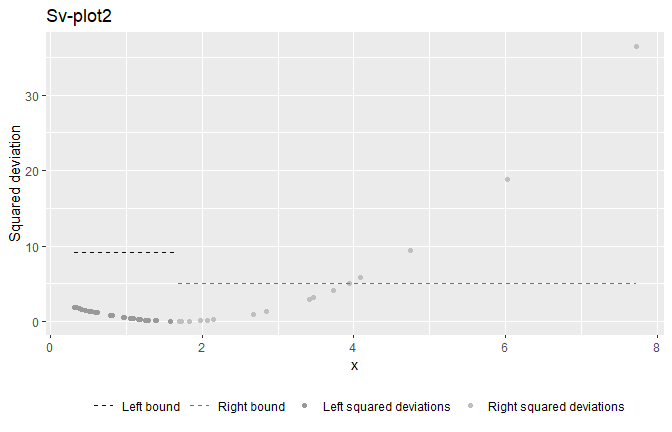Figure 3. Longer curve length traced by points from average to the farthest point in right signals that the data follow a right skewed distribution. Five dots above the right dash line are squared deviations corresponding to outliers.

### Example 4

set.seed(10)
X<-matrix(rnorm(50,mean=8,sd=2))
svplot2(X,title="Sv-plot2",xlab="x",lbcol="grey5",lscol="grey60",rbcol="grey45",rscol="grey75")
#> $Summary #> Sample_Size Average Left_SS Right_SS Sample_Variance #> 1 50 7.3174 77.9674 69.1623 3.0026 #> #>$Svplot2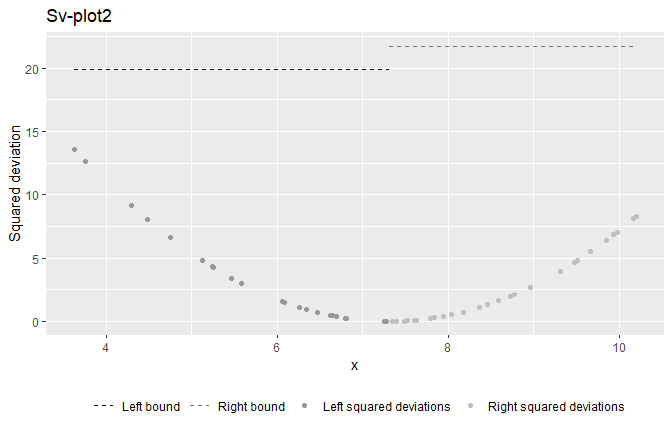Figure 4. Approximately equal curve lengths traced by points from average to the farthest points in left and right appears that the data follow a symmetric distribution. This dataset is lack of outliers.

## Testing Hypotheses by Sv-plot2

As an innovative graphical method, Sv-plot2 can be used to make the decision in hypothesis testing. To illustrate Sv-plot2 with both data and summary statistics, the entire graph of the upward parabola traced by the squared deviations is used as Sv-plot2 in hypothesis testing. In examples 5 through 11, significance level is set to be 5%.

### Testing Hypotheses for Single Population Mean

Two Sv-plots2s created at the sample average and hypothesized mean along with the horizontal dash line created at squared half of the margin of error, are placed on the same plot. If the intersection point is on or above the horizontal line, the null hypothesis is rejected at the specified significance level, otherwise, fail to reject the null hypothesis. Examples 5 and 6 display use of test1mu function as graphical tool to make the decision on testing hypothesis over single mean based on two simulated datasets whereas Example 7 displays use of test1musm function based on summary statistics.

#### Example 5 (Small sample with unknown sigma)

set.seed(5)
X=matrix(rnorm(20,mean=3,sd=2))
test1mu(X,mu0=3.5,alpha=0.05,unkwnsigma=TRUE,sigma=NULL,xlab="x",
title="Single mean: Hypothesis testing by Sv-plot2",
samcol="grey5",popcol="grey45",thrcol="black")
#> $Summary #> Sample_Size Average Stdev Intersection_Point Decision_by_Svplot2 pvalue #> 1 20 2.4389 1.8588 Above the threshold Reject H_0 0.0194 #> #>$Svplot2s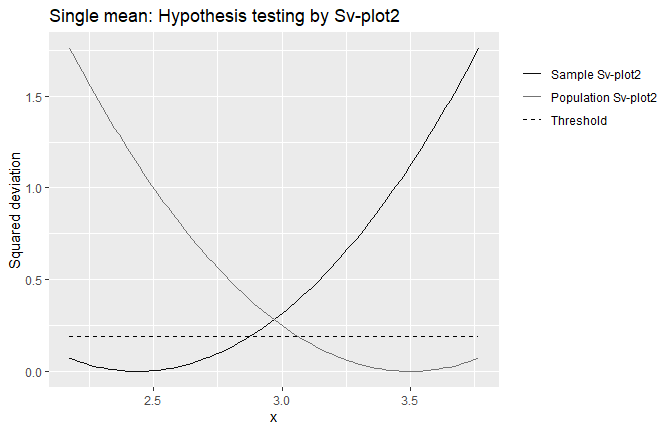Figure 5. The intersection point lies above the dash line, and hence reject the null hypothesis that the population mean is 3.5 at 5% significance level.

#### Example 6 (Large sample with known sigma)

set.seed(5)
X=matrix(rnorm(40,mean=3,sd=2))
test1mu(X,mu0=3.5,alpha=0.05,unkwnsigma=FALSE,sigma=2,xlab="x",
title="Single mean: Hypothesis testing by Sv-plot2",
samcol="grey5",popcol="grey45",thrcol="black")
#> $Summary #> Sample_Size Average Stdev Intersection_Point Decision_by_Svplot2 pvalue #> 1 40 3.1357 2.2023 Below the threshold Fail to reject H_0 0.2493 #> #>$Svplot2s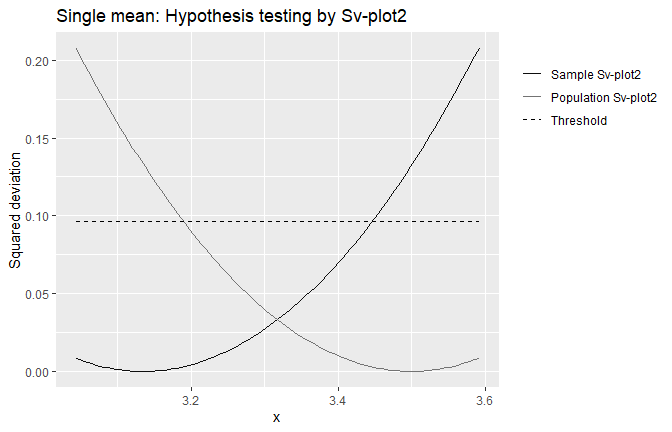Figure 6. The intersection point lies below the dash line, and hence fail to reject the null hypothesis that the population mean is 3.5.

#### Example 7

     test1musm(n=20,xbar=3,s=2,mu0=4.5,alpha=0.05, unkwnsigma=TRUE,sigma=NULL,xlab="x",
title="Single mean summary: Hypothesis testing by Sv-plot2",
samcol="grey5",popcol="grey45",thrcol="black")
#> $Summary #> Intersection_Point Decision_by_Svplot2 pvalue #> 1 Above the threshold Reject H_0 0.0033 #> #>$Svplot2s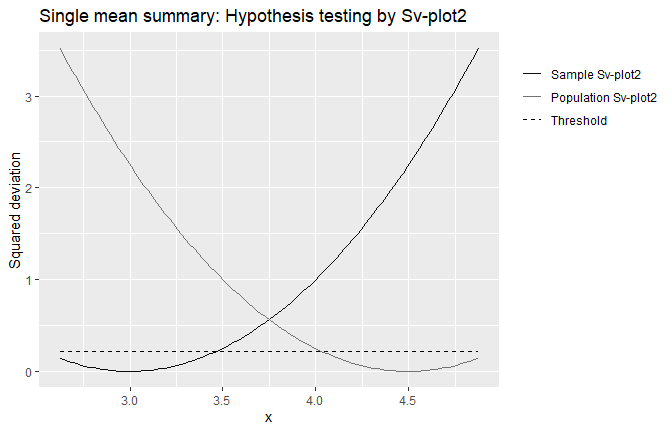Figure 7. The intersection point lies above the dash line, and hence reject the null hypothesis that the population mean is 4.5 at 5% significance level.

### Testing Hypotheses for two Population Means

Two Sv-plots2s created at the sample averages and threshold horizontal line created squaring the half of the margin of error will be displayed on the same plot and make the decision as described for single population mean. Examples 8 displays use of test2mu function to make the decision on testing hypothesis over two means based on two independent simulated datasets whereas Example 8 displays that from two dependent samples.

#### Example 8 (Small samples with unknown sigma)

set.seed(5)
test2mu(X1=matrix(rnorm(10,mean=3,sd=2)),X2=matrix(rnorm(20,mean=4,sd=2.5)),
paired=FALSE,eqlvar=FALSE,unkwnsigmas=TRUE,
sigma1=NULL,sigma2=NULL,alpha=0.05,
sam1col="grey5",sam2col="grey45",thrcol="black")
#> $Summary #> Sample Size Average Stdev Intersection_Point Decision_by_Svplot2 pvalue #> 1 1 10 2.8423 1.9047 Below the threshold Fail to reject H_0 0.1327 #> 2 2 20 4.1409 2.5794 #> #>$Svplot2s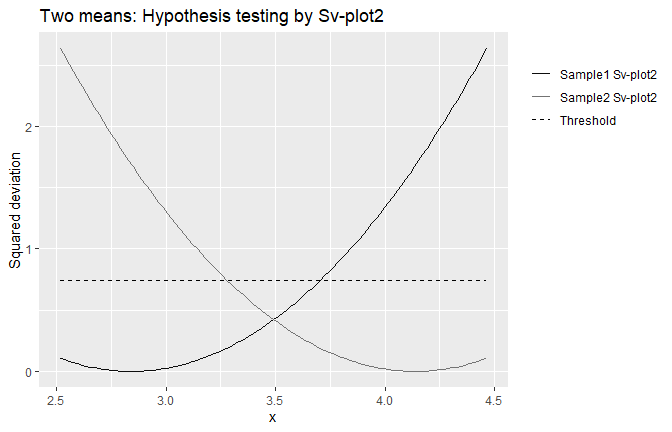Figure 8. The intersection point lies below the dash line, and hence fail to reject the null hypothesis that the population means equal.

#### Example 9

set.seed(5)
X1=matrix(rnorm(10,mean=4,sd=2))
X2=2*X1
test2mu(X1,X2,
paired=FALSE,eqlvar=FALSE,unkwnsigmas=TRUE,
sigma1=NULL,sigma2=NULL,alpha=0.05,
sam1col="grey5",sam2col="grey45",thrcol="black")
#> $Summary #> Sample Size Average Stdev Intersection_Point Decision_by_Svplot2 pvalue #> 1 1 10 3.8423 1.9047 Above the threshold Reject H_0 0.0134 #> 2 2 10 7.6846 3.8093 #> #>$Svplot2s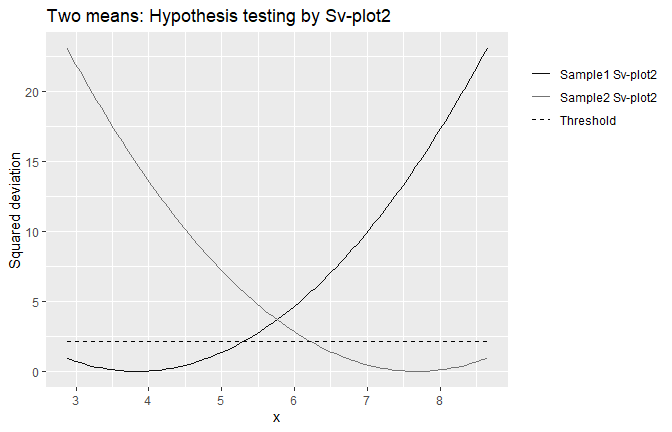Figure 9. The intersection point lies above the dash line, and hence reject the null hypothesis that the population means equal at 5% significance level.

Examples 10 displays use of test2musm function to make the decision of testing hypothesis on two means based on summary statistics from two independent samples whereas Example 10 displays that from two dependent samples.

#### Example 10

test2musm(n1=20,n2=25,xbar1=3,xbar2=4,s1=1,s2=1.5,
paired=FALSE,eqlvar=FALSE,unkwnsigmas=TRUE,
sigma1=NULL,sigma2=NULL,sdevdif=NULL,alpha=0.05,
xlab="x",title="Two means summary: Hypothesis testing by Sv-plot2",
sam1col="grey5",sam2col="grey45",thrcol="black")
#> $Summary #> Intersection_Point Decision_by_Svplot2 pvalue #> 1 Above the threshold Reject H_0 0.0107 #> #>$Svplot2s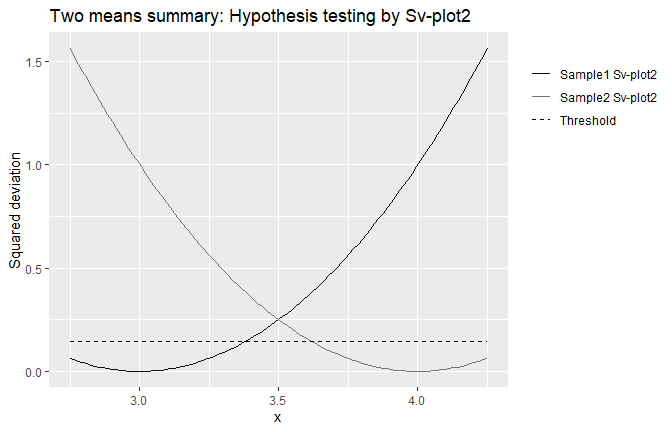Figure 10. The intersection point lies above the dash line, and hence reject the null hypothesis that the population means equal at 5% significance level.

#### Example 11

test2musm(n1=20,n2=25,xbar1=3,xbar2=3.7552127,s1=1,s2=1.5,
paired=FALSE,eqlvar=FALSE,unkwnsigmas=TRUE,
sigma1=NULL,sigma2=NULL,sdevdif=NULL,alpha=0.05,
xlab="x",title="Two means summary: Hypothesis testing by Sv-plot2",
sam1col="grey5",sam2col="grey45",thrcol="black")
#> $Summary #> Intersection_Point Decision_by_Svplot2 pvalue #> 1 On the threshold Reject H_0 0.05 #> #>$Svplot2s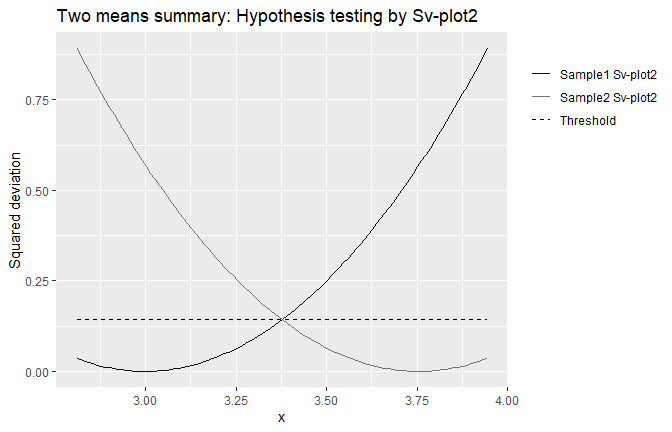Figure 11. The intersection point lies on the dash line, and hence reject the null hypothesis that the population means equal at 5% significance level.

## Reference

Wijesuriya, Uditha Amarananda. 2020. “Sv-Plots for Identifying Characteristics of the Distribution and Testing Hypotheses.” Communications in Statistics-Simulation and Computation. https://doi.org/10.1080/03610918.2020.1851716.